# Convolutional Neural Networks

Posted by Xiaozhe Yao on May 31, 2020

# Overview

Convolutional neural network (CNN or ConvNet) is a class of deep neural networks, which plays an important role in image classification. Though there are several different types of CNNs, they have similar architectures. From the aspect of different functions, their common components can be categorized into the following:

• Convolution and Pooling Layers. They begin with several stacks of convolution and pooling layers. Usually, we periodically insert a pooling layer in-between successive convolution layers in a ConvNet architecture. These stacks convert the vector into a high-dimensional feature map, i.e. these stacks are trying to get easy-to-classify features.
• Fully Connected Layers. After several stacks of convolution and pool- ing layers, there will be several fully-connected layers. These layers are used to classify images into different categories.
• Softmax Layer. At the end of the CNNs, there will be a softmax layer which converts the results of fully connected layers into probabilities of each category.

After these layers, we see that an image will be converted into a list of probabilities of each category. In the following part of this section, we will first explain how each layer works in more detail, and then investigate an example, the VGG net.

## Convolutional Layers

With the above image representation, we can perform convolution operation on the tensor. The Convolution layer’s parameters consist of a set of learnable filters, or called kernels. The filters are spatially small, and with the same depth with the input data. When performing the convolution operation, we slide (more precisely, convolve) the filters across the width and height of the input data, and compute dot products between the entries of the filter and the input at every position. As we slide the filter over the width and height of the input, we will produce a 2-dimensional matrix that gives the responses of that filter at every spatial position. The sliding process is illustrated in Fig 1.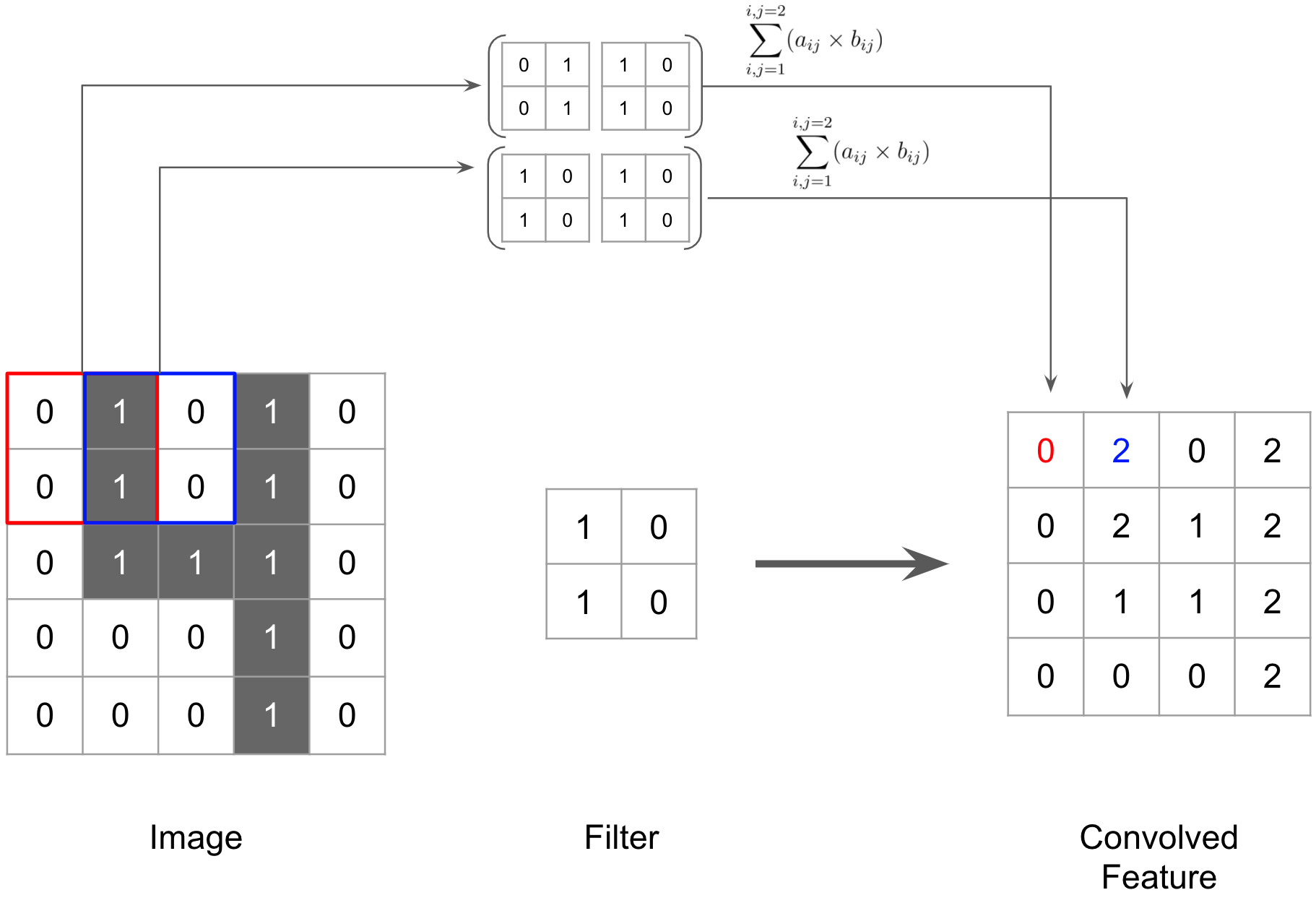Fig.1 Illustration of Convolution Operation with the kernel size of 2 × 2 × 1 on a 5 × 5 × 1 image. There are two boxes on the left upper corner as examples. After these two computations, we continue to slide the filter to the right corner. After the first two rows, we then slide on the second and third row. We will keep sliding the filter again and again until we go through the whole image. After the sliding process, we will get a 4 × 4 matrix.

Each filter can be regarded as a pattern extractor. By using a manually- chosen filter, we can extract some high-level features from an image, for example, boundaries. In Fig 2, we demonstrate the high-level features after an edge detec- tion filter. Thus, the key idea behind filter is that, by learning the parameters in filters, we can have several high-level features from the original image, and achieve higher classification accuracy with these features.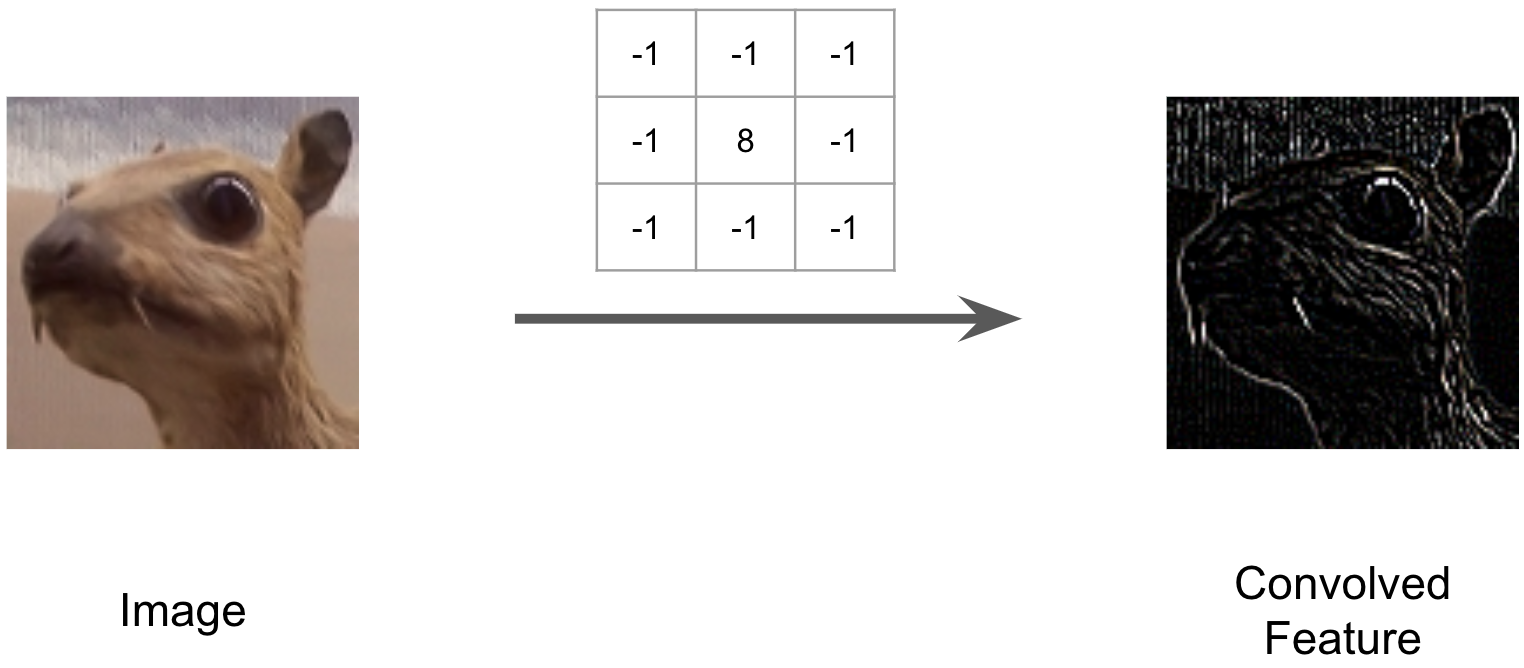Fig.2 An example of a filter works as a feature extractor. In this example, we apply a manually chosen filter to an image, and get the boundaries of the object in the image.

From the computation process of convolution operation, we see that the depth of filters in the first convolution layer must be the same with the depth of the input image. If it is not the case, we will not be able to compute the dot product. Then, the output of convolution operation, no matter the depth of the original image, is always a 2-dimensional matrix because the output of the dot product is a scalar. This 2-dimensional matrix is called feature map or activation map.

In each layer, we can have multiple filters stacking together to extract different features. They are independent of each other, and we simply stack them into a single tensor to give the output. Therefore, the output of the convolution layer is also a tensor with width, height and depth, here the depth is the number of filters. To summarize, Convolution layers are used as feature extractor, and we want it to extract some highly classifiable features so that our classification algorithm easily recognize these features. In deep learning, we do not manually set the parameters in a filter, but we initialize them with random values, and then find a good one (it’s usually not the global optimal one, and even not the local optimal, so it is only acceptable) during the training.

## Pooling Layers

Pooling layer is another building block of a CNN. Like filters, pooling layer also has pooling kernel with a width and height, and it basically chooses an element as output in the kernel. The process is illustrated in Fig 3. Pooling layer aims to progressively reduce the spatial size of the intermediate tensors to reduce the number of parameters and computation in the network. Pooling layers do not need any parameters to compute.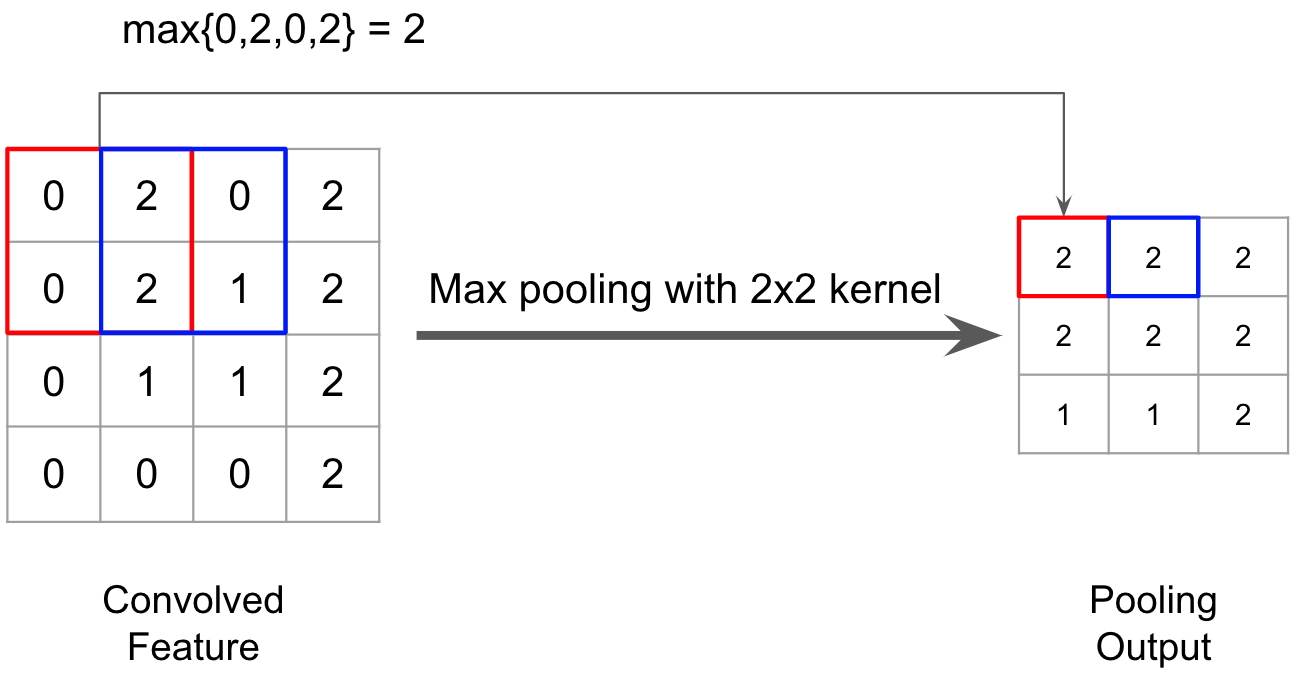Fig.3 Illustration of max pooling layer with a 2 × 2 kernel.

There are also some other kinds of poolings, such as average pooling, which returns the average value as output. In practice, people found that max pooling usually works better.

Pooling layer operates independently on every depth slice of the input. The output of a pooling layer is also a 3-dimensional tensor, with the same depth as the last layer, but a smaller width and height.

## Fully Connected Layers

Fully connected layers in convolutional neural networks are the same with regu- lar neural networks. The input to the first fully connected layer is the output of pooling layer. More precisely, the output of the pooling layer, as a 3-dimensional tensor, will be first flattened to a vector, and then fed into the fully connected layers. In Fig 4, we illustrated a structure of several fully connected layers.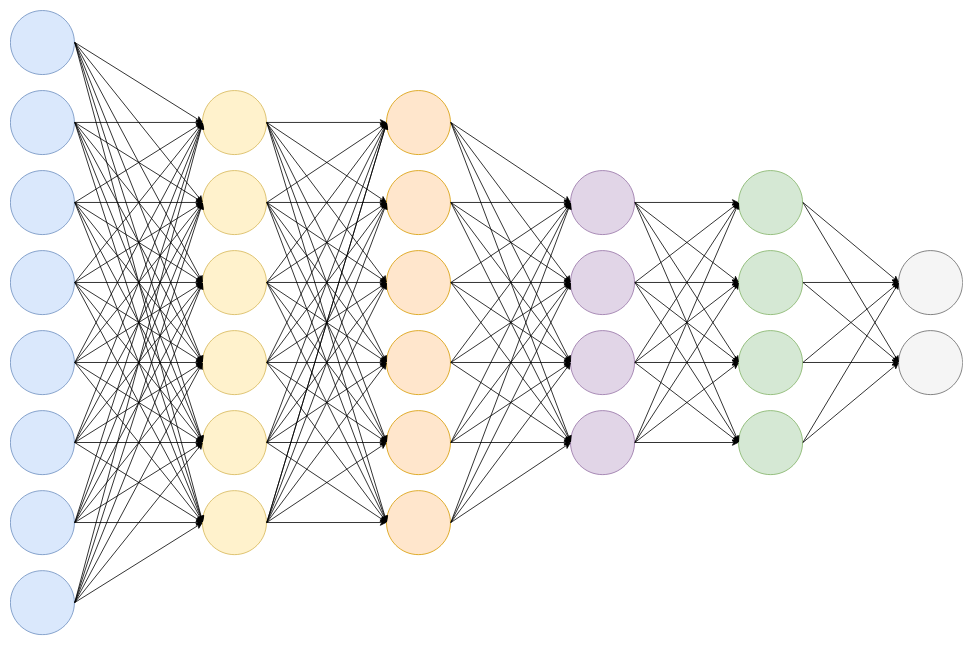Fig.4 Illustration of several fully connected layers.

In each layer, we perform the computation as $f(X) = g(w^TX + b)$, where $w$ (for weight) and $b$ (for bias) are parameters that we learned during the model training and g is an activation function. If we assume that layer $i$ requires a $p_i \times 1$ vector as input, and its output is $q_i$, then the weight will be a $p_i \times q_i$ matrix and the bias will be a $q_i \times 1$ vector. The weight and bias will be learned during the training process.

After computing the $w^TX + b$, we usually apply an activation function on the result. The purpose of such functions is to add some non-linearity to the neural networks. As we see above, all operations we have are linear. Images in real life are usually not linear, and thus it cannot be approximated by such a linear system. To tackle the problems, we add some non-linear functions at the end of each fully connected layer. The most successful one is ReLU(Rectified Linear Unit) function, which basically equals to $g(x) = max(0, x)$.

After the fully connected layers, we will have $n$ nodes where $n$ equals the number of categories. Each of them is a non-negative value.

## Softmax Layer

At the end of our neural networks, we want our output to be a probability dis- tribution. Thus we apply softmax function to each node. The softmax function is defined as: $$\delta(z_i)=\frac{e^{z_i}}{\sum_{j=1}^{n}e^{z_j}}$$ where $n$ represents the number of categories.

At the end of the whole neural network, we get a list of probabilities of each category. As we see in the above process, to perform the image classification, the convolution layer and fully connected layers require some parameters to compute the output. These parameters will be computed in the model training process by using gradient descent approach. After the training process, these parameters will be saved to a weight file. When performing inference, we just load the parameters from the file into memory, and compute the output of the neural networks.

# VGG Net

With these backgrounds in the convolutional neural network, the VGG net becomes simple. In the original paper of VGG net, they call the convolution layer and fully connected layer as weight layer, as they require the parameters. There are two types of VGG Net in their paper: VGG-16 and VGG-19. They both have three the same fully connected layers, thus VGG-16 has 13 convolution layers and VGG-19 has 16 convolution layers.

To simplify the illustration, we will use VGG-16 as an example. The architecture of VGG-16 is illustrated in Fig 5. In the illustration, we see that it requires the input to be a 224 × 224 × 3 tensor, and then perform convolution operation and max pooling. After several convolution and max pooling layers, we will have a high-level feature map of that image, and the output will be fed into three fully connected layers for classification. In the end, there will be 1000 nodes as the final output, since there are 1000 categories in the original dataset (the imagenet dataset) used by VGG net.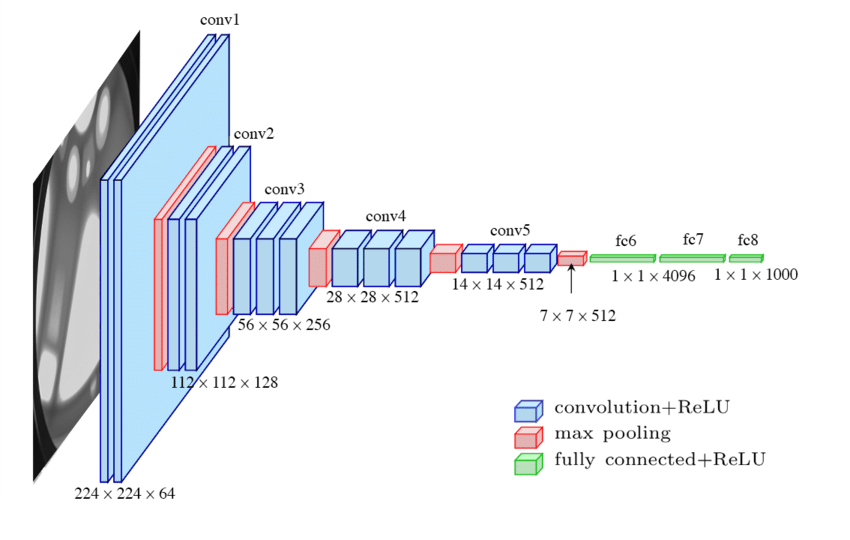Fig.5 Illustration of VGG-16 Architecture.

The contribution of VGG net is that it shows the depth of the neural network is a critical component for good performance. It has a much deeper network architecture and much more parameters (138 million) than others at that time. As it has a rather clear architecture, but are expensive to evaluate, it becomes a good starting point for other researches, such as model serving, model compression, etc.

From our previous understanding of convolution layers and fully connected layers, we can understand that the first 13 weight layers, i.e. the convolution layers work as the feature extractor while the last three weight layers, i.e. the fully connected layers work as the classifier. One thing that needed to be pointed out here is that, after training with the large imagenet dataset, we can remove the parameters for the last 3 fully connected layers, and only remain the parameters for the first 13 convolution layers. Then if we need to build an image classification algorithm on a new dataset, we can fix the parameters for the first 13 convolution layers, and only compute new parameters for the last 3 fully con- nected layers. This process is called Transfer Learning.